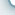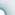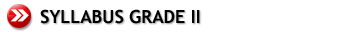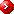Primary Mathematics U.S. Edition 2ANumbers to 1000Addition and SubtractionLength Looking Back Comparing Numbers Hundreds, Tens and Ones Meanings of Addition and Subtraction Addition Without Renaming Subtraction Without Renaming Addition With Renaming Subtraction With Renaming Measuring Length in Meters Measuring Length in Centimeters Measuring Length in Yards and Feet Measuring Length in InchesWeight Multiplication and Division Multiplication Tables of 2 and 3 Measuring Weight in Kilograms Measuring Weight in Grams Measuring Weight in Pounds Measuring Weight in Ounces Multiplication Division Multiplication Table of 2 Multiplication Table of 3 Dividing by 2 Dividing by 3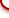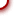Primary Mathematics U.S. Edition 2BAddition and SubtractionMultiplication and DivisionMoney (U.S. currency) Finding the Missing Number Methods for Mental Addition Methods for Mental Subtraction Multiplying and Dividing by 4 Multiplying and Dividing by 5 Multiplying and Dividing by 10 Counting Dollars and Cents Adding Money Subtracting MoneyFractions Time Capacity Halves and Quarters Writing Fractions Telling Time Time Intervals Comparing Capacity Liters Gallons, Quarts, Pints and CupsGraphs Geometry Area Picture Graphs Flat and Curved Faces Making Shapes Square UnitscybeRShala Difference Lesson Illustration How It Works Compare Us Request Info Placement Test Tell a friend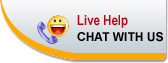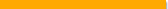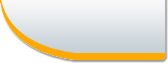Home | FAQ | Tell a Friend | Contact Us | Compare Us | Homeschool Resources | Sitemap cybershala.com Inc. All Rights reserved | Terms of service | Privacy policy